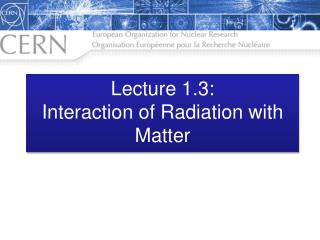DownloadDownload PresentationLecture 1.3: Interaction of Radiation with Matter

# Lecture 1.3: Interaction of Radiation with Matter

Download Presentation## Lecture 1.3: Interaction of Radiation with Matter

- - - - - - - - - - - - - - - - - - - - - - - - - - - E N D - - - - - - - - - - - - - - - - - - - - - - - - - - -
##### Presentation Transcript

1. Lecture 1.3:Interaction of Radiation with Matter

2. Outline 1. Energy loss by heavy particles 2. Multiple scattering through small angles 3. Photon and Electron interactions in matter Radiation Length Energy loss by electrons Critical Energy Energy loss by photons Bremsstrahlung and pair production 4. Electromagnetic cascade 5. Muon energy loss at high energy 6. Cherenkov and Transition Radiation

3. Electromagnetic Interaction of Particles with Matter If particle’s velocity is greater than the speed of light in the medium -> Cherenkov Radiation. When crossing the boundary between median, ~1% probability of producing a Transition Radiation X-ray. Interaction with atomic nucleus. Particle undergoes multiple scattering. Could emit a bremsstrahlung photon. Interaction with atomic electrons. Particle loses energy; atoms are excited or ionized.

4. Cross-section Material with atomic mass A and density ρ contains n atoms A volume with surface S and thickness dx contains N=nSdx atoms S dx Probability of incoming particle hitting an atom Probablity that a particle hits exactly one atom between x and (x + dx) Ave collisions/cm Mean free path

5. Differential Cross-section Differential cross-section is the cross-section from an incoming particle of energy E to lose an energy between E and E’ Total cross-section Probability (P(E)) that a particle of energy, E, loses between E’ and E’ + dE’ in a collision Average number of collisions/cm causing an energy loss between E’ and E’+dE’ Average energy loss per cm

6. Stopping Power Linear stopping power (S) is the differential energy loss of the particle in the material divided by the differential path length. Also called the specific energy loss. Stopping Power of muons in copper Bethe-Bloch Formula Particle Data Group Energy loss through ionization and atomic excitation

7. Range Integrate the Bethe-Bloch formula to obtain the range. Useful for low energy hadrons and muons with momenta below a few hundred GeV Radiative Effects important at higher momenta. Additional effects at lower momenta.

8. Photon and Electron Interactions in Matter Electrons: bremsstrahlung e Presence of nucleus required for the conservation of energy and momentum γ γ e p p n n p p n n p p n n p p n n p p p p n n p p n n n n Photons: pair production Characteristic amount of matter traversed for these interactions is the radiation length (X0) e e

9. Radiation Length Energy Loss in Lead Mean distance over which an electron loses all but 1/e of its energy through bremsstralung But also 7/9 of the mean free path for electron-positron pair production by a high energy photon

10. Energy Loss by Electrons A charged particle of mass M and charge q=Z1e is deflected by a nucleus of charge Ze (charge partially shielded by electrons) The deflection accelerates the charge and therefore it radiates bremsstrahlung Elastic scattering of a nucleus is described by Partial screening of nucleus by electrons

11. Electron Critical Energy Energy loss through bremsstrahlung is proportional to the electron energy Ionization loss is proportional to the logarithm of the electron energy Critical energy (Ec) is the energy at which the two loss rates are equal Electron in Copper: Ec = 20 MeV Muon in Copper: Ec = 400 GeV!

12. Photon Energy Loss Contributing Processes Light element:Carbon Atomic photoelectric effect Rayleigh scattering Compton scattering of an electron Pair production (nuclear field) Pair production (electron field) Photonuclear interaction (Giant Dipole Resonance) Heavy element:Lead At low energies the photoelectric effect dominates; with increasing energy pair production becomes increasingly dominant.

13. Photon Pair Production Probability that a photon interaction will result in a pair production Differential Cross-section Total Cross-section

14. Electromagnetic Cascades Longitudinal Shower Profile A high-energy electron or photon incident on a thick absorber initiates an electromagnetic cascade through bremsstrahlung and pair production Longitudinal development scales with the radiation length Electrons eventually fall beneath critical energy and then lose further energy through dissipation and ionization Measure distance in radiation lengths and energy in units of critical energy

15. Electromagnetic Cascades Visualization of cascades developing in the CMS electromagnetic and hadronic calorimeters

16. Electromagnetic Cascades Transverse shower size scales with the Molière radius On average 10% of the shower energy lies outside a cylinder with radius RM. About 99% within 3.5RM.

17. Muon Energy Loss For muons the critical energy (above which radiative processes are more important than ionization) is at several hundred GeV. Pair production, bremsstrahlung and photonuclear Ionization energy loss Mean range

18. Muon Energy Loss Critical energy defined as the energy at which radiative and ionization energy losses are equal. Muon critical energy for the chemical elements

19. Muon Tomography Luis Alvarez used the attenuation of muons to look for chambers in the Second Giza Pyramid He proved that there are no chambers present

20. From Interactions to Detectors Now that you know all the interactions, we can start talking about detectors!